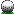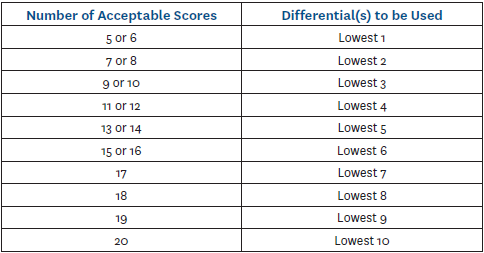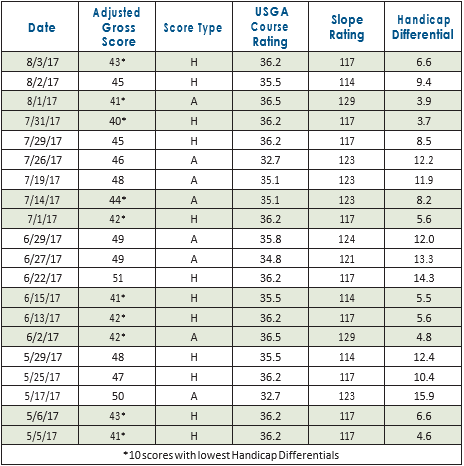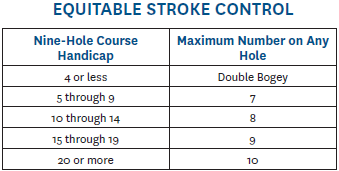HomeHandicap calculation The site uses the pre-2020 USGA Handicap formula to compute handicaps. The formula computes a handicap index which is converted to a handicap using the course slope. The maximum handicap index is 18.2 which converts to a maximum Village Greens front nine handicap of 19.5 Village Greens Woodridge  front-9 white: par: 36, slope: 121, rating: 35.2  back-9 white: par: 36, slope: 127, rating: 35.0  * you can find slopes for other tees in the USGA National Course DatabaseCourse Handicap is calculated as      Course Handicap = Handicap Index * (Slope of Tee on Course / 113)e.g. Player has handicap index of 6.0 and plays the back-9 white tees at Village Greens (slope: 127)      6.0 * (127/113) = 6.7 (rounded to nearest tenth) The handicap index formula uses a certain number of round scores (based on the table in the USGA manual). For example, if you have 5 or 6 rounds the formula uses 1 (best) score. For 7 or 8 rounds it uses 2 best scores. The maximum number of scores used is 10 when you have 20 acceptable scores. The score used in the calculation is an adjusted gross score (AGS) which may be less than the actual score due to maximum scores per hole based on the player's course handicap. For example if a player has a course handicap of 4 or less the maximum score per hole is double-bogey for handicap calculation.One local rule we use is everyone (with at least one round played) gets a handicap index. This is so we have a handicap for all players to compute standings and points. We use only the 1 best score until the player reaches 7 rounds.*** the following is from the USGA Handicap manual 10-5. Nine-Hole Handicaps-Handicap Index (N) a. Nine-Hole Handicap Use and Identification A nine-hole handicap is a Handicap Index (N) if the club follows the USGA Handicap System. A nine-hole handicap, or Handicap Index (N), may be used in inter-club play against other players with nine-hole handicaps. If a competition requires a Handicap Index, a Handicap Index (N) should be doubled for 18-hole play (See Decision 3-2/1 and 3-2/2). A player with both a Handicap Index and a Handicap Index (N) should use the Handicap Index (N) in inter-club nine-hole competition and the Handicap Index in 18-hole competition. A player with only a Handicap Index playing in a nine-hole competition should halve the Handicap Index and round the decimal upward to the nearest tenth, then converts it to a Course Handicap using the nine-hole Slope Rating from the Course Handicap Table for the tees being played. b. Method of Computation Golf clubs compute a Handicap Index (N) by applying nine-hole adjusted gross scores to the USGA Course Rating and Slope Rating of the nine holes played to determine Handicap Differentials. The procedure for calculating the Handicap Index (N) is as follows: Step 1: Use the table below to determine the number of Handicap Differentials to use: Note our league uses 1 differential when a player has 6 or less acceptable scores.Step 2: Determine the Handicap Differential(s); Step 3: Average the Handicap Differential(s) being used; Step 4: Multiply the average by .96*; Step 5: Delete all numbers after the tenths' digit (truncate). Do not round to the nearest tenth. Example 1: Fewer than 20 scores (11 scores available).   Total of 4 lowest Handicap Differentials: 34.5   Average (34.5 / 4): 8.625   Average multiplied by .96: 8.28   Delete all digits after tenths digit (truncate). Do not round to the nearest tenth: 8.2   Handicap Index (N): 8.2 Example 2: The following is an example of a Handicap Index (N) calculation for a player with 20 scores available.   Total of 10 lowest Handicap Differentials: 55.1   Average (55.1 / 10): 5.51   Average multiplied by .96: 5.289   Delete all digits after tenth: 5.2   Handicap Index (N): 5.2c. Nine-hole Equitable Stroke Control See definition of Equitable Stroke Control.Example: A player with a nine-hole Course Handicap of 12 must adjust to a maximum score of 8 for any hole, regardless of par. d. Maximum Nine-Hole Handicap Index The maximum Handicap Index (N) is 18.2 for men and 20.2 for women. Note : A maximum Handicap Index will convert to a Course Handicap that exceeds these numbers on tees with a Slope Rating greater than 113.